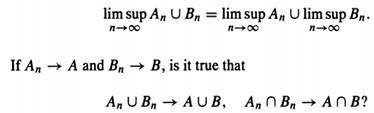### Create an Account

Already have account?

### Forgot Your Password ?

Home / Questions / Suppose Ω = 0 1 and C = 0 Enumerate n. the class of all a-fields containing C Suppose Ω ...

# Suppose Ω = 0 1 and C = 0 Enumerate n. the class of all a-fields containing C Suppose Ω = 0 1 2 and C =0 Enumerate n. the class of all σ-fields containing C and give

Suppose Ω = {0, 1} and C = {(0}}. Enumerate n. the class of all a-fields containing C.

2. Suppose Ω = (0, 1, 2} and C = ( (0} }. Enumerate n. the class of all σ-fields containing C and give a (C).

3. Let An. A, Bn, B be subsets of Ω. ShowJun 22 2020 View more View Less

#### Answer (Solved)Subscribe To Get Solution